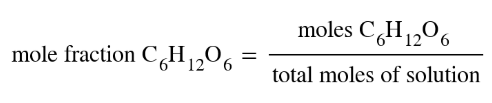# Problem: In lab you need to prepare at least 100 mL of each of the following solutions. Explain how you would proceed using the given information. d. 0.10 mole fraction of C6H12O6 in water (d = 1.00 g/cm3)

###### FREE Expert Solution
• Recall that mole fraction is equal to:• Total moles of solution is equal to the moles of water and moles of glucose

Moles of solution = moles water + moles glucose

• We can get the the moles of C6H12O6 , eventually the mass, using the provided volume of solution
87% (393 ratings)###### Problem Details

In lab you need to prepare at least 100 mL of each of the following solutions. Explain how you would proceed using the given information.

d. 0.10 mole fraction of C6H12O6 in water (d = 1.00 g/cm3)